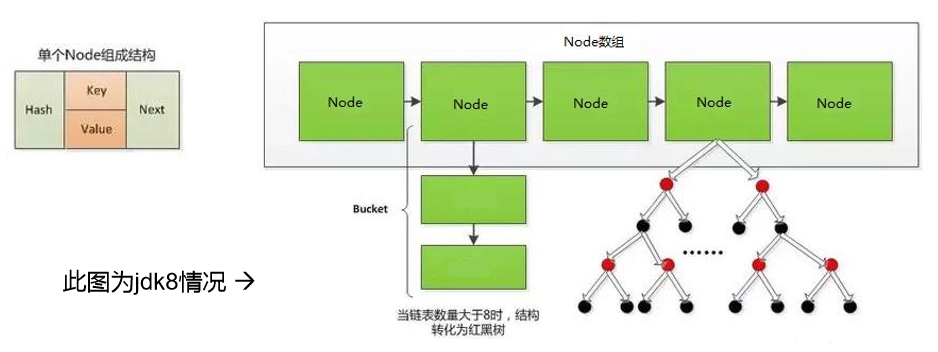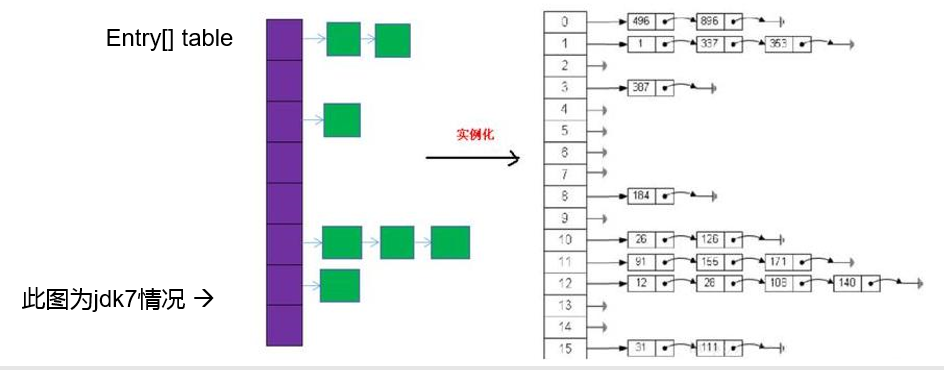# 彻底搞懂jdk1.8中的haspMap原理（源码解析+ 对比jdk1.7）

#### 二、HashMap的底层实现原理

##### jdk1.8

HashMap的大致结构如下图所示：其中哈希表是一个Node数组，我们把数组中可以存放元素的位置称为一个桶（Bucket），哈希表每个节点都有一个键值（Key–value）对，hash值和Next指针，在插入元素时，如果发生冲突（即多个键值对映射到同一个桶上），就会通过单向链表来解决冲突。因为一个桶上可能存在多个键值对，所以在查找的时候，会先通过key的哈希值定位到桶，在遍历桶上的所有键值对，找出key相等的键值对，从而找到value。HashMap什么时候进行扩容和树形化

##### jdk1.7

jdk1.7中存取节点的不是Node 而是Entry，Node和Entry中的元素都一样（hash key value next）。它的底层结构是数组+单向链表，它实例化之后底层创建了一个默认长度为16的数组#### 三 源码解析

##### 1、属性：
     /**
* 默认初始化容量为16
*/
static final int DEFAULT_INITIAL_CAPACITY = 1 << 4;

/**
* 最大的容量上限为2的30次方
*/
static final int MAXIMUM_CAPACITY = 1 << 30;

/**
* 默认的负载因子是0.75f
*/
static final float DEFAULT_LOAD_FACTOR = 0.75f;

/**
* 变成树形结构的临界值为8
*/
static final int TREEIFY_THRESHOLD = 8;

/**
* 当哈希表的大小超过这个阈值，才会把链式结构转化成树型结构，否则仅采
*取扩容来尝试减少冲突
*/
static final int MIN_TREEIFY_CAPACITY = 64;

/**
* 哈希表
*/
transient Node<K,V>[] table;

/**
* 哈希表中键值对的个数
*/
transient int size;

/**
* 哈希表被修改的次数
*/
transient int modCount;

/**
*它是通过 capacity*loadFactor 计算出来的，当 size 到达这个值时，
*就会进行扩容操作
*/
int threshold;

/**
*负载因子
*/
final float loadFactor;


Node类的定义，它是一个静态内部类，哈希表中的每一个节点都是Node类型。我们可以看到，Node中有四个属性key、value、hash（存储key的hash值）、next（构建链表的时候用来指向后继节点的），后边我们存储不能重复的key，所以hashcode 和equals是重写的。

    static class Node<K,V> implements Map.Entry<K,V> {
final int hash;
final K key;
V value;
Node<K,V> next;

Node(int hash, K key, V value, Node<K,V> next) {
this.hash = hash;
this.key = key;
this.value = value;
this.next = next;
}

public final K getKey()        { return key; }
public final V getValue()      { return value; }
public final String toString() { return key + "=" + value; }

public final int hashCode() {
return Objects.hashCode(key) ^ Objects.hashCode(value);
}

public final V setValue(V newValue) {
V oldValue = value;
value = newValue;
return oldValue;
}

public final boolean equals(Object o) {
if (o == this)
return true;
if (o instanceof Map.Entry) {
Map.Entry<?,?> e = (Map.Entry<?,?>)o;
if (Objects.equals(key, e.getKey()) &&
Objects.equals(value, e.getValue()))
return true;
}
return false;
}
}

##### 3、get方法：
public class HashMap<K,V> extends AbstractMap<K,V>
implements Map<K,V>, Cloneable, Serializable {

//返回值类型为V,HashMap<K,V>中的V
public V get(Object key) {
Node<K,V> e;
return (e = getNode(hash(key), key)) == null ? null : e.value;
}

final Node<K,V> getNode(int hash, Object key) {
Node<K,V>[] tab; Node<K,V> first, e; int n; K k;
// 如果hash表不为空且对应的桶不为空，其中的tab[(n - 1) & hash]中的&是进行按位与操作
if ((tab = table) != null && (n = tab.length) > 0 &&
(first = tab[(n - 1) & hash]) != null) {
//如果hash值相等且key值也相等，表示取到值
if (first.hash == hash && // always check first node
((k = first.key) == key || (key != null && key.equals(k))))
return first;
//判断是否由后继节点
if ((e = first.next) != null) {
// 如果当前的桶是采用红黑树处理冲突，则调用红黑树的 get 方法去获取节点
if (first instanceof TreeNode)
return ((TreeNode<K,V>)first).getTreeNode(hash, key);
//不是红黑树的话，那就是传统的链式结构了，通过循环的方法判断链中是否存在该 key
do {
if (e.hash == hash &&
((k = e.key) == key || (key != null && key.equals(k))))
return e;
} while ((e = e.next) != null);
}
}
return null;
}
}


1、通过 hash 值获取该 key 映射到的桶。
2、桶上的 key 就是要查找的 key，则直接命中。
3、桶上的 key 不是要查找的 key，则查看后续节点：
(1）如果后续节点是树节点，通过调用树的方法查找该 key。
（2）如果后续节点是链式节点，则通过循环遍历链查找该 key。

##### 4、put 方法
    public V put(K key, V value) {
return putVal(hash(key), key, value, false, true);
}

final V putVal(int hash, K key, V value, boolean onlyIfAbsent,
boolean evict) {
Node<K,V>[] tab; Node<K,V> p; int n, i;
//如果哈希表为空，则先创建一个哈希表（resize里面实现了初始化 大小为默认值16）
if ((tab = table) == null || (n = tab.length) == 0)
n = (tab = resize()).length;
//如果当前桶没有碰撞冲突，则直接把键值对插入
if ((p = tab[i = (n - 1) & hash]) == null)
tab[i] = newNode(hash, key, value, null);
else {
Node<K,V> e; K k;
//如果桶上节点的 key 与当前 key 重复，那你就是我要找的节点了，表示的是更新
if (p.hash == hash &&
((k = p.key) == key || (key != null && key.equals(k))))
e = p;
//如果是采用红黑树的方式处理冲突，则通过红黑树的 putTreeVal 方法去插入这个键值对
else if (p instanceof TreeNode)
e = ((TreeNode<K,V>)p).putTreeVal(this, tab, hash, key, value);
//否则就是传统的链式结构
else {
//采用循环遍历的方式，判断链中是否有重复的 key
for (int binCount = 0; ; ++binCount) {
//到了链尾还没找到重复的 key，则说明 HashMap 没有包含该键 ，添加
if ((e = p.next) == null) {
//创建一个新节点插入到尾部
p.next = newNode(hash, key, value, null);
//如果链的长度大于 TREEIFY_THRESHOLD（默认为8） 这个临界值，则调用treeifBin方
//法，注意不一定生成红黑树，下面对 treeifyBin有解释
if (binCount >= TREEIFY_THRESHOLD - 1) // -1 for 1st
treeifyBin(tab, hash);
break;
}
//找到了重复的 key ，中断
if (e.hash == hash &&
((k = e.key) == key || (key != null && key.equals(k))))
break;
p = e;
}
}
//这里表示在上面的操作中找到了重复的键，所以这里把该键的值替换为新值
if (e != null) { // existing mapping for key
V oldValue = e.value;
if (!onlyIfAbsent || oldValue == null)
e.value = value;
afterNodeAccess(e);
return oldValue;
}
}
++modCount;
//判断是否需要进行扩容
if (++size > threshold)
resize();
afterNodeInsertion(evict);
return null;
}

final void treeifyBin(Node<K,V>[] tab, int hash) {
int n, index; Node<K,V> e;
//如果当前数组不是null 或者数组的大小小于MIN_TREEIFY_CAPACITY（默认64），则进行扩容
if (tab == null || (n = tab.length) < MIN_TREEIFY_CAPACITY)
resize();
//否则改为红黑树
else if ((e = tab[index = (n - 1) & hash]) != null) {
TreeNode<K,V> hd = null, tl = null;
do {
TreeNode<K,V> p = replacementTreeNode(e, null);
if (tl == null)
hd = p;
else {
p.prev = tl;
tl.next = p;
}
tl = p;
} while ((e = e.next) != null);
if ((tab[index] = hd) != null)
hd.treeify(tab);
}
}


put 方法比较复杂，实现步骤大致如下：
1、先通过 hash 值计算出 key 映射到哪个桶。
2、如果桶上没有碰撞冲突，则直接插入。
3、如果出现碰撞冲突了，则需要处理冲突： （
1）如果该桶使用红黑树处理冲突，则调用红黑树的方法插入。
2）否则采用传统的链式方法插入。如果链的长度到达临界值（默认8）且当前的数组的长度>64时，此时此索引位置上的所有的数据改为红黑树存储
4、如果桶中存在重复的键，则为该键替换新值。
5、如果size 大于阈值，则进行扩容。

#### 四、长问面试题

##### 2、谈谈你对HashMap中put/get方法的认识？如果了解再谈谈 HashMap的扩容机制？默认大小是多少？什么是负载因子( 或填充比)？什么是吞吐临界值(或阈值、threshold)？

03-121万+10-112万+
01-078万+
08-022万+
05-253万+
04-237万+
04-2499
07-0846
07-301250
11-0542万+
©️2020 CSDN 皮肤主题: 代码科技 设计师:Amelia_0503